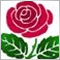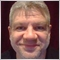# How strategy tester profit is calculated?284

Hi,

could you tell me how strategy tester calculates profit?.

Regards,

Vince.1101

I'd guess that it's (Exit Price - Entry Price) * Position Size * Pip Value.25849

```profitInAccountCurrency = ( OrderClosePrice() - OrderOpenPrice() )* Direction( OrderType() )
* OrderLots() * DeltaValuePerLot( OrderSymbol() ) ;```284

Thanks enivid...

WHRoeder, i am working with a index instrument, i guess i have to manually set the profit, maybe in an excel, because with indexes i have a ticksize and tickvalue (a little different than forex), any idea?

Regards,

Vince.284

How can show the correct profit in strategy tester?.

For instance,  the instrument (cfd index) that i am testing has this properties:

Digits: 2

Tick value: 2.50

Tick size: 0.25

Regards,

Vince.284

Sorry, was my fault,, the result is correct i had set USD insted of EUR in the testing tab, damn.

Regards,

Vince.284

Problems again, is this a bug?

TickValue (full lot) = 2.50

TickSize = 0.25

EUR Account, Look the profit in Strategy Tester

1) 3730.25 - 3715.50  =  14.75 / 0.25 = 59 ticks * 2.50 = 147.50 -> 147.50 CORRECT

2) 3721.50 - 3711.50 =  10 / 0.25 =  40 ticks * 2.50 = 100 -> 96.13 WRONG!!! Why calculates 96.13?

What's happening?

Regards,

Vince.42595

coiler:

Problems again, is this a bug?

TickValue (full lot) = 2.50

TickSize = 0.25

EUR Account, Look the profit in Strategy Tester

1) 3730.25 - 3715.50  =  14.75 / 0.25 = 59 ticks * 2.50 = 147.50 -> 147.50 CORRECT

2) 3721.50 - 3711.50 =  10 / 0.25 =  40 ticks * 2.50 = 100 -> 96.13 WRONG!!! Why calculates 96.13?

What's happening?

Regards,

Vince.

Swap is happening. Your second trade close on a next day, so you pay the swap.